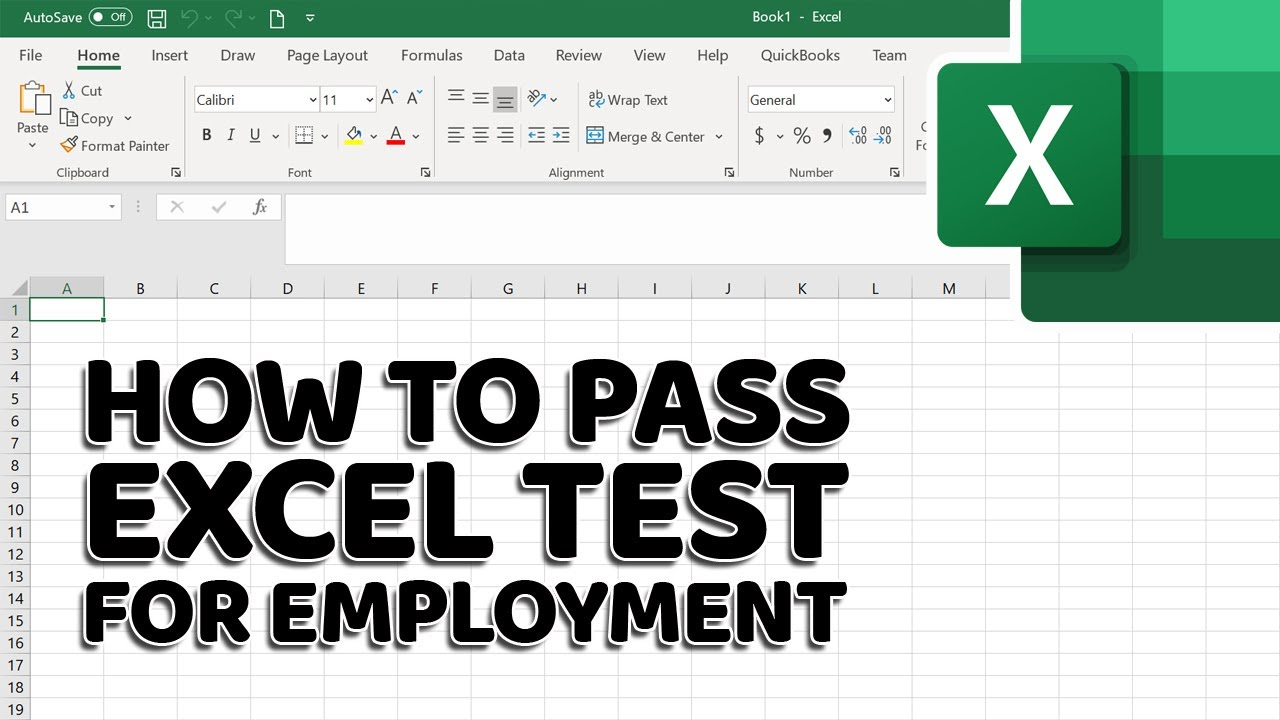## How to Pass Excel Assessment Test For Job Applications – Step by Step Tutorial with XLSX work files

Get ready for Excel interview Assessment Test for job application using this step by step guide. In this tutorial you will learn 20 typical assessment exercises, asked during Excel interview assessment test. SUBSCRIBE!!! https://www.howtoanalyzedata.net/youtube

In This Video:
1. How to extend Column B to fit Total Cost by month in Excel 00:01
2. How to calculate Total Cost of expenses by month using Formula in Excel 02:11
3. How to create a border around the table in Excel 03:43
4. How to format the data as currency in Excel 04:25
5. How to use a formula to calculate Total Costs in Excel 05:08
6. How to use a formula to calculate Average Costs in Excel 06:30
7. How to change the alignment in column D to right in Excel 07:51
8. How to calculate quarterly cost for Q1 (Quarter 1) and Q2 in Excel 08:13
9. How to save the file to Documents Folder in Excel 09:13
10. How to change page orientation to Landscape in Excel 09:40
11. How to fit work table into single page for print out in Excel 10:06
12. How to center table header values in Excel 11:47
13. How to check the spelling of the document in Excel 12:06
14. How to rename Sheet1 as Business Expenses in Excel 12:58
15. How to add a new worksheet in Excel 13:35
16. How to create a column chart to show expenses for the first quarter in Excel 13:52
17. How to change the width of Columns I and J so the contents fits in Excel 15:20
18. How to bold all headings and change headings font to 12 points in Excel 15:46
19. How to merge and Center the table heading “Business Expenses” in Excel 16:25
20. How to forecast Lease cost for third quarter by calculating Q3 total in Excel 17:19
__________
LETS CONNECT!!!
Subscribe to Email: https://www.howtoanalyzedata.net/email

Other Recommended Excel Videos:
The Beginner’s Guide to Excel – Excel Basics Tutorial: https://www.youtube.com/watch?v=rwbho0CgEAE
Microsoft Excel Tutorial for Beginners – Full Course: https://www.youtube.com/watch?v=Vl0H-qTclOg
Microsoft Excel Tutorial – Beginners Level 1: https://www.youtube.com/watch?v=k1VUZEVuDJ8
Master the MOST POPULAR Excel Formulas and Functions:https://www.youtube.com/watch?v=eBkMQxIIkx4
Excel Basics 1: Introduction To Excel 1: Formatting, Formulas, Cell References, Page Setup: https://www.youtube.com/watch?v=c_ZJLJK5PjM

__________
Other Online Training Resources: https://www.howtoanalyzedata.net/resources/

__________
Excel Test for Job Interview Videos